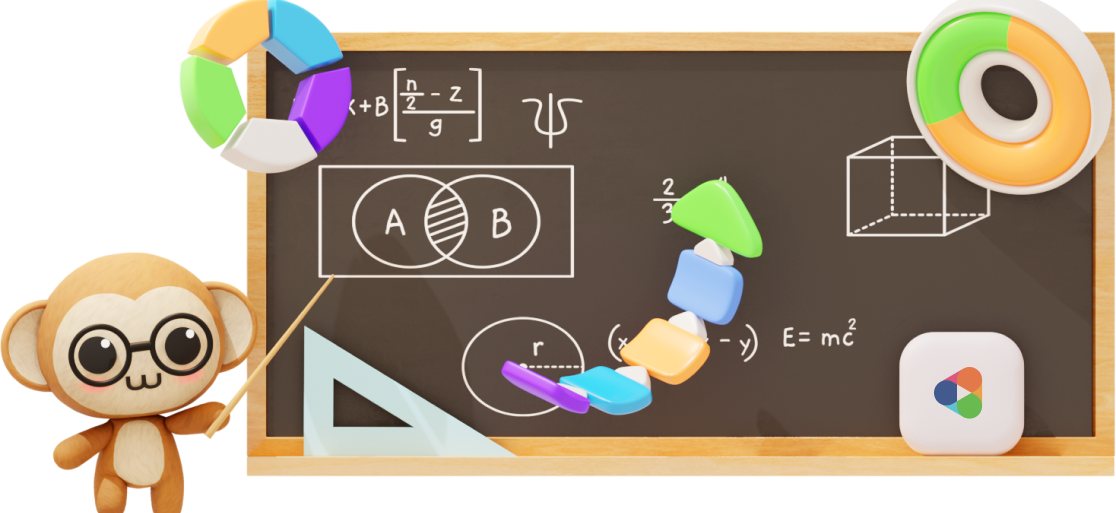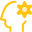# Primary 6 Syllabus

Aligned with the latest 2021 Singapore MOE Math syllabus and endorsed by Education Alliance of Finland.
Hundreds of hours of learning content for all topics covered in P6 to get your child build a strong Math foundation!## Overview

Many parents and children tend to stress out at this point.

Fear not — Practicle is here!

Our question bank comprises questions from top school exam papers to help you get the practice you need with the bonus of expanding your collection of virtual pets and rewards in our system! Revision doesn’t have to be boring all the time!

Start practicing now!

### Features##### Gamification to make learning engaging and rewarding##### A.I. + Practicle system to personalise questions to your child’s ability### Overview of Primary 6 PSLE Math Syllabus

The PSLE Math syllabus consist of topics learnt in Primary 5 and Primary 6 as PSLE is a 2-year course for students.

The 2021 Primary 6 PSLE Math Syllabus can be divided into 3 main branches:

• Number and Algebra
• Measurement and Geometry
• Statistics

#### P6 PSLE Math: Numbers and Algebra

The Primary 6 (P6) topics that are covered under Number and Algebra are Algebra, Whole Number, Fraction, Decimal, Percentage, Ratio and Speed, Rate and Time. Refer below for the breakdown of the skills in each topic.#### Whole Numbers

• Notate & Represent Place Values Up to 10 000
• Read and Write Numbers in Words Up to 1 Mil
• Apply Order of Operations
• Divide by 10, 100, 1000 & their Multiples
• Multiply with 10, 100, 1000 & their Multiples
• Solve Problem Sums Involving 4 Operations#### Algebra

• Notate & Interpret Simple Algebraic Expressions
• Solve Simple Equations
• Solve Simple Linear Equations
• Solve Simple Linear Expressions by Substitution
• Form & Solve Simple Linear Equations in Problem Sums#### Fractions

• Divide Whole Number by Proper Fraction
• Divide Proper Fraction by Proper Fraction
• Divide Proper Fraction by Whole Number
• Solve Fraction Problem Sums#### Ratio

• Understand the Relationship Between Fractions & Ratio
• Find Ratio of 2 Quantities in Direct Proportion
• Solve Problem Sums Involving 3 Quantities
• Solve Problem Sums that Involves Changing Ratio#### Percentage

• Find the Whole given a Part of the Percentage
• Find Percentage Increase/Decrease
• Solve Problem Sums Involving Percentages#### Decimals*

• Round Off Decimals to the Nearest Whole Number, 1 Decimal or 2 Decimal Places
• Multiply Decimals up to 2-digit Whole Number
• Divide Decimals up to 2-digit Whole Number
• Multiply Decimals by 10, 100 1000 and their Multiples
• Divide Decimals by 10, 100 1000 and their Multiples
• Convert Measurements of Length, Mass & Volume
• Solve Problem Sums Involving 4 Operations#### Speed

• Write Speed in Different Units
• Find Time, given Distance and Speed
• Find Speed, given Time and Distance
• Find Distance, given Time and Speed
• Solve Problem Sums Involving Speed
• Differentiate between Speed & Average Speed

#### P6 PSLE Math: Measurement and Geometry

The Primary 6 (P6) topics that are covered under Measurement and Geometry are Area and Perimeter, Volume, Angles, Shapes and Properties, 2D/3D Representation and Nets. Refer to the following for the breakdown of the skills in each topic.#### Circles

• Find Diameter of Circle, given Radius & Vice Versa
• Estimate Area of Circle Using a Square Grid
• Find Area/Circumference of Circle
• Find Area/Perimeter of Semi Circle
• Find Area/Perimeter of Quarter Circle
• Find Area of Composite Figures
• Find Perimeter of Composite Figures#### Volume

• Find 1 Dimension of Cuboid, given Volume & Other Dimensions
• Find Length of 1 Edge of Cube, given Volume
• Find Height of Cuboid, given Volume and Base Area
• Find Area of a Face of Cuboid, given Volume and 1 Dimension
• Solve Problem Sums Involving Volume of Liquid#### Angles

• Find Unknown Angles in Composite Figures#### 2D/3D Shapes

• Associate 2-D Drawings with 3-D Solids
• Visualize Prisms, Cylinders, Pyramids & Cones from Drawings
• Draw 2-D Representations of Solids
• Convert measurements of length, mass & volume
• Identify the Solid that can be Formed by a Given Net
• Identify the Net that can be Used to Form a Given Solid

#### P6 PSLE Math: Statistics

The Primary 6 (P6) topics that are covered under Statistics include Average and Data Analysis. Refer below for the breakdown of the skills in each topic.

Although the students do not have the topic on Average (denoted by *) in their P6 textbook, it will still be tested at the PSLE level.#### Average*

• Find the Average, given Total Value & the Number of Data
• Find the Total Value, given the Average & the Number of Data
• Solve Problem Sums Involving Average#### Data Analysis

• Solve Problems Using Info from Pie Charts

### Sample P6 questions and video explanations### Experience Practicle free, no commitment no hidden cost no lock-in

Try our learning platform free with a 7 day trial
and see if your child likes it.